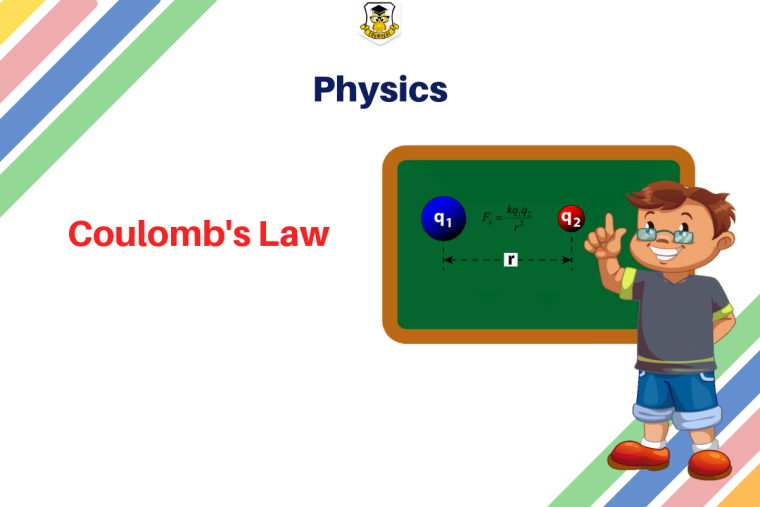## Eduwhere's Concept: Coulomb's Law### Coulomb’s law statement:

The electrostatic force of attraction or repulsion between two point charges is directly proportional to the product of charges. The electrostatic force of attraction or repulsion between two point charges is inversely proportional to the square of the distance between them.

Let’s consider, there are two charges q1 and q2. The distance between both these charges is ‘r’, and the force of attraction/repulsion between them is ‘F’. At this time,

F  ∝ q1q2

Or, F  ∝  1/r2

F  = k q1q2/ r2

here k is a proportionality constant and equals to 1/4 π ε0. Here, ε0 is the epsilon naught which signifies permittivity of a vacuum. The value of k comes 9 × 109 Nm2/ C2 when we take the S.I unit of value of ε0 is 8.854 × 10-12 C2 N-1 m-2.

Let’s also take a look at the Vector Form of Coulomb’s Law

### Vector Form of Coulomb’s Law

The physical quantities are of two types namely scalars (with the only magnitude) and vectors (those quantities with magnitude and direction). Force is a vector quantity because it has both magnitude and direction. Coulomb’s law can be re-written in the form of vectors. Remember we denote the vector “F” as F, vector r as r and so on.

Let there be two charges q1 and q2, with position vectors r1 and r2 respectively. Now, since both the charges are of the same sign, there will be a repulsive force between them. Let the force on the q1 charge due to q2 be F12 and force on q2 charge due to q1charge be F21. The corresponding vector from q1 to q2 is r21 vector.

r21 = r2 – r1

To denote the direction of a vector from position vector r1 to r2, and from r2 to r1 as:Now, the force on charge q2 due to q1, in vector form is:The above equation is the vector form of Coulomb’s Law.

1

2511